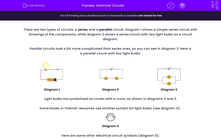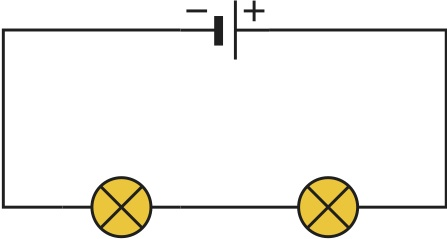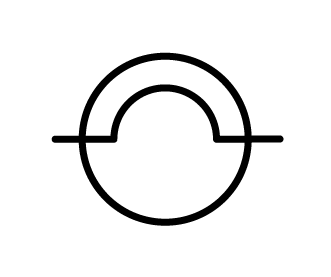# Recognise Circuit Symbols in Series and Parallel Circuits

In this worksheet, students will follow an introduction to electrical circuits.Key stage:  KS 3

Curriculum topic:   Physics: Electricity and Electromagnetism

Curriculum subtopic:   Current Electricity

Popular topics:   Physics worksheets, Electricity worksheets

Difficulty level:#### Worksheet Overview

There are two types of circuits: a series and a parallel circuit. Diagram 1 shows a simple series circuit with drawings of the components, while diagram 2 shows a series circuit with two light bulbs as a circuit diagram.

Parallel circuits look a bit more complicated than series ones, as you can see in diagram 3. Here is a parallel circuit with two light bulbs.Diagram 1 Diagram 2 Diagram 3

Light bulbs are symbolised as circles with a cross, as shown in diagrams 2 and 3.

Some books or Internet resources use another symbol for light bulbs (see diagram 4).Diagram 4

Here are some other electrical circuit symbols (diagram 5):Diagram 5

Wires are simply represented as lines.

In a series circuit, all light bulbs light together and if one breaks, a gap is created in the circuit, so none of them works. In a parallel circuit, the current flows along all branches. If one light bulb is broken, the others can still work. Christmas lights are connected in parallel. No one wants their Christmas lights off, because of just one bulb!

In a series circuit, adding more bulbs would make all of them dimmer, because this would increase the resistance in the circuit. In a parallel circuit, though, if you add more branches with bulbs in them, they will all still be bright.

Current is the same everywhere in a series circuit, but it splits up in branches in a parallel circuit. In the latter, current in all branches adds up to the current in the main branch.

### What is EdPlace?

We're your National Curriculum aligned online education content provider helping each child succeed in English, maths and science from year 1 to GCSE. With an EdPlace account you’ll be able to track and measure progress, helping each child achieve their best. We build confidence and attainment by personalising each child’s learning at a level that suits them.

Get started